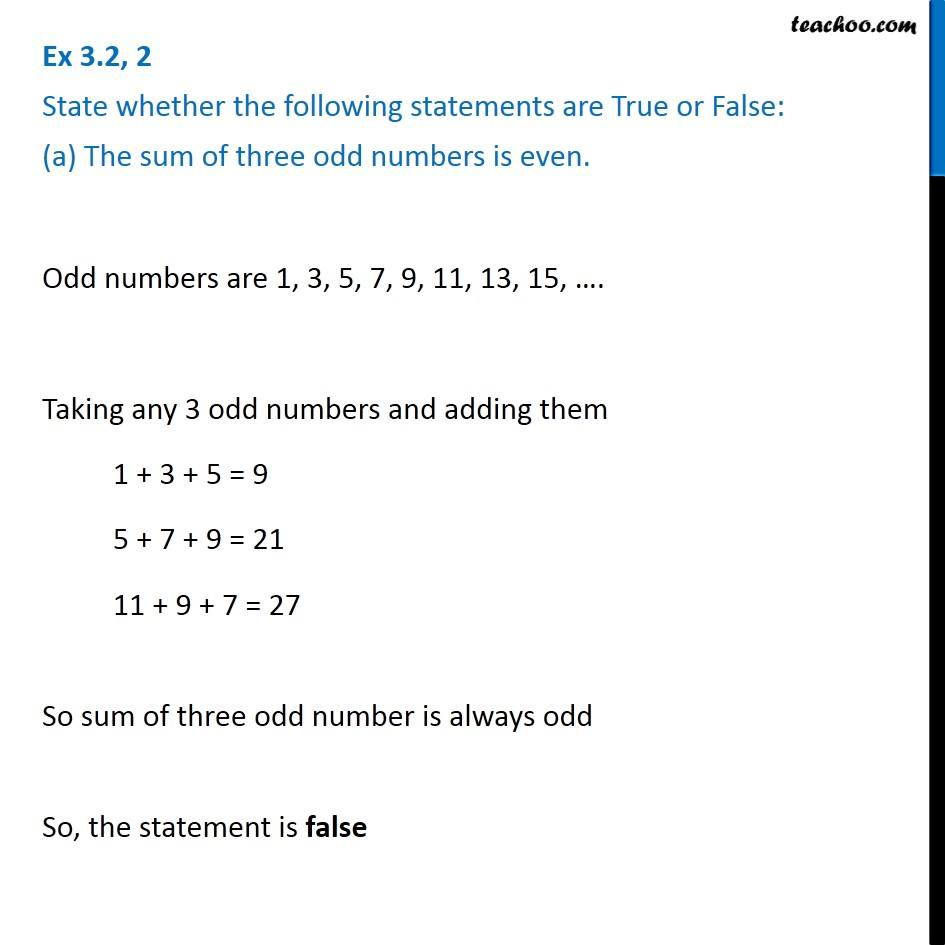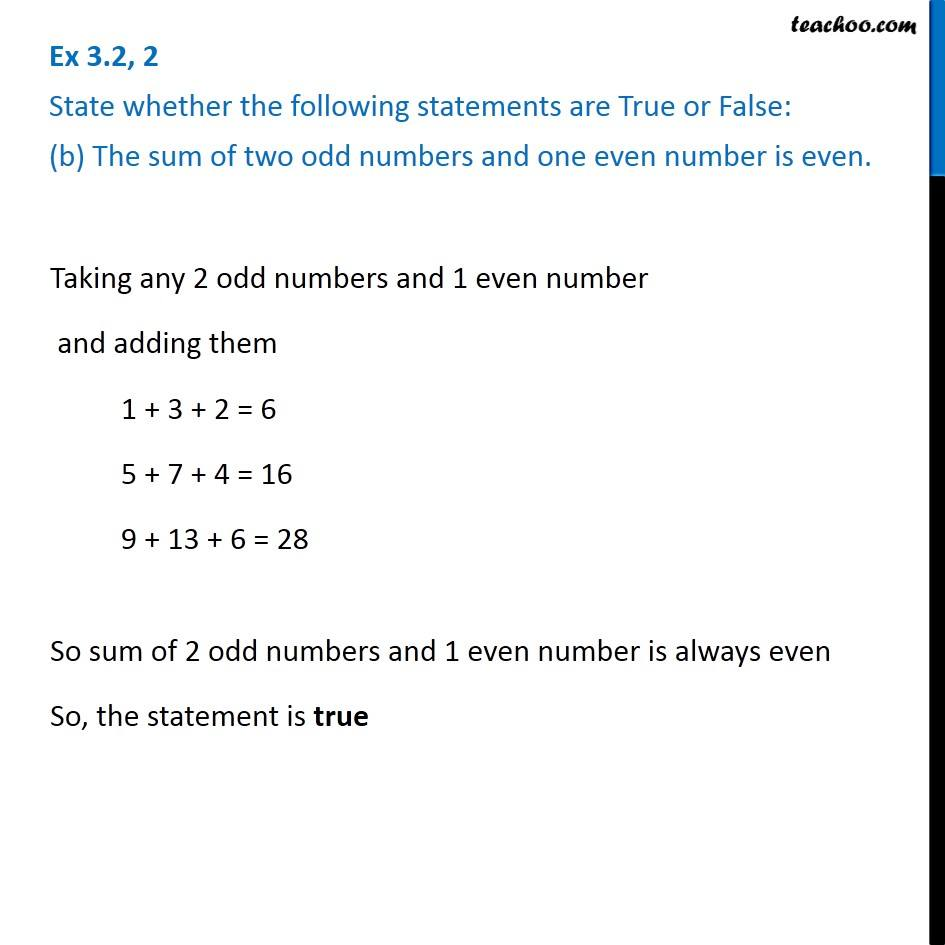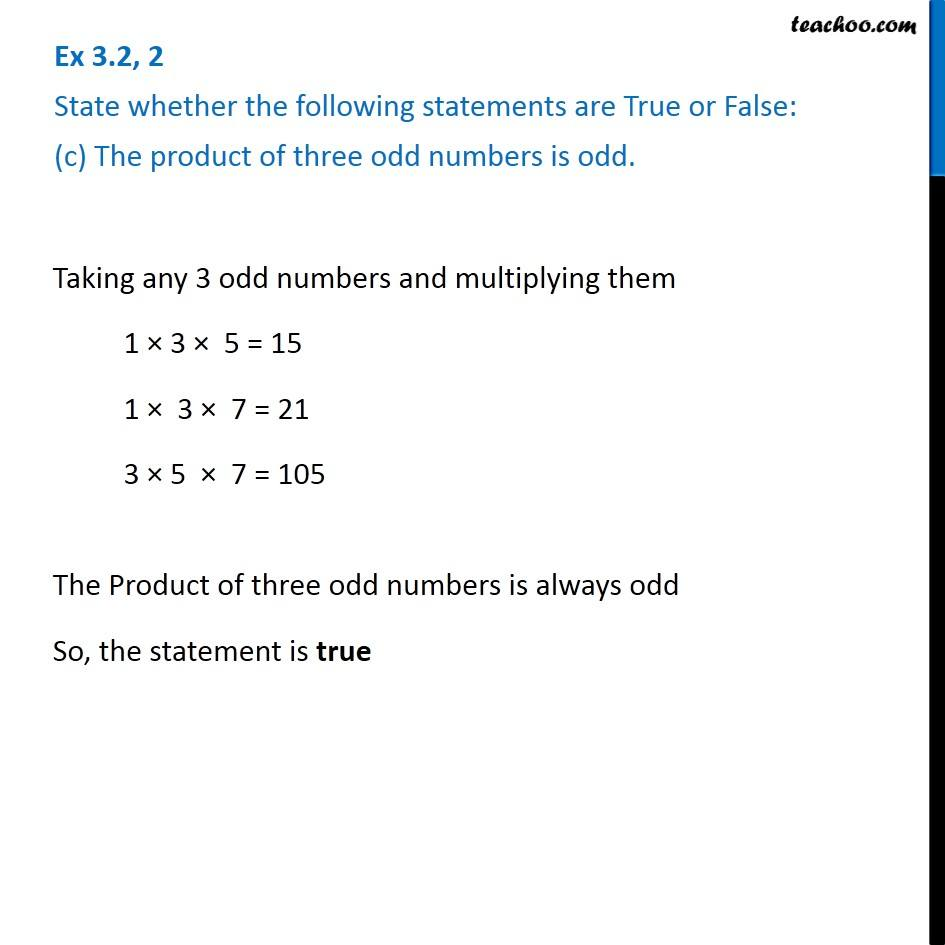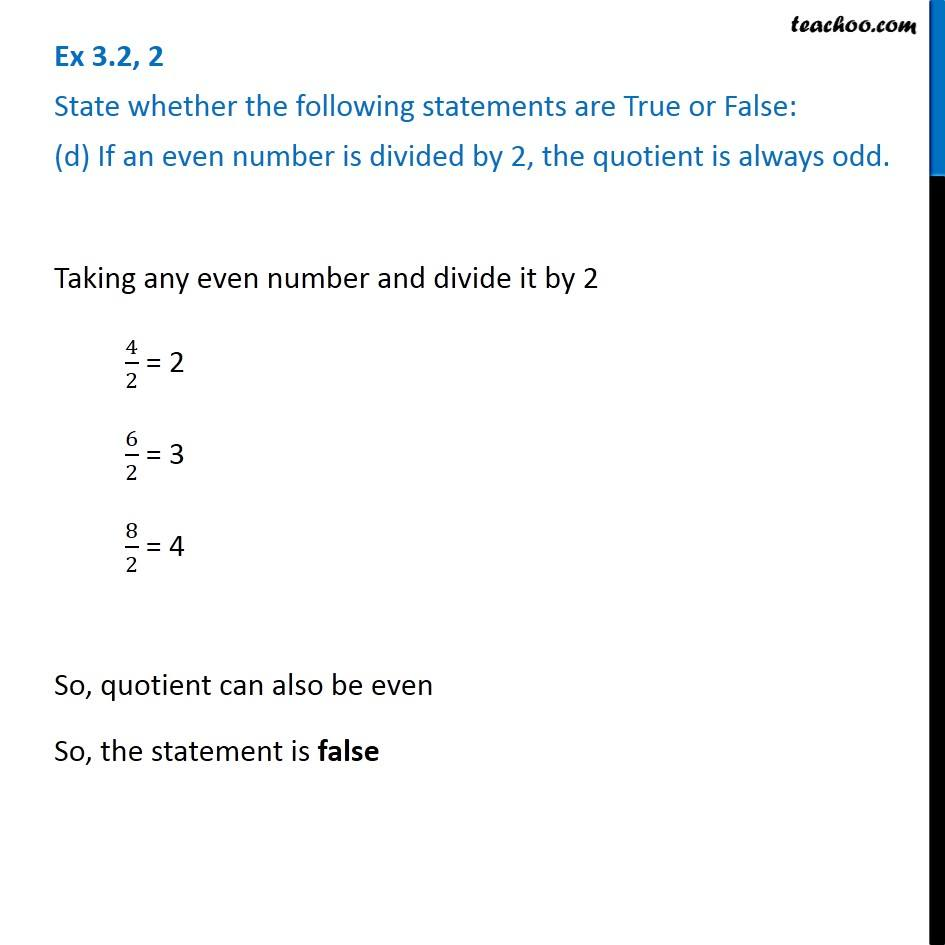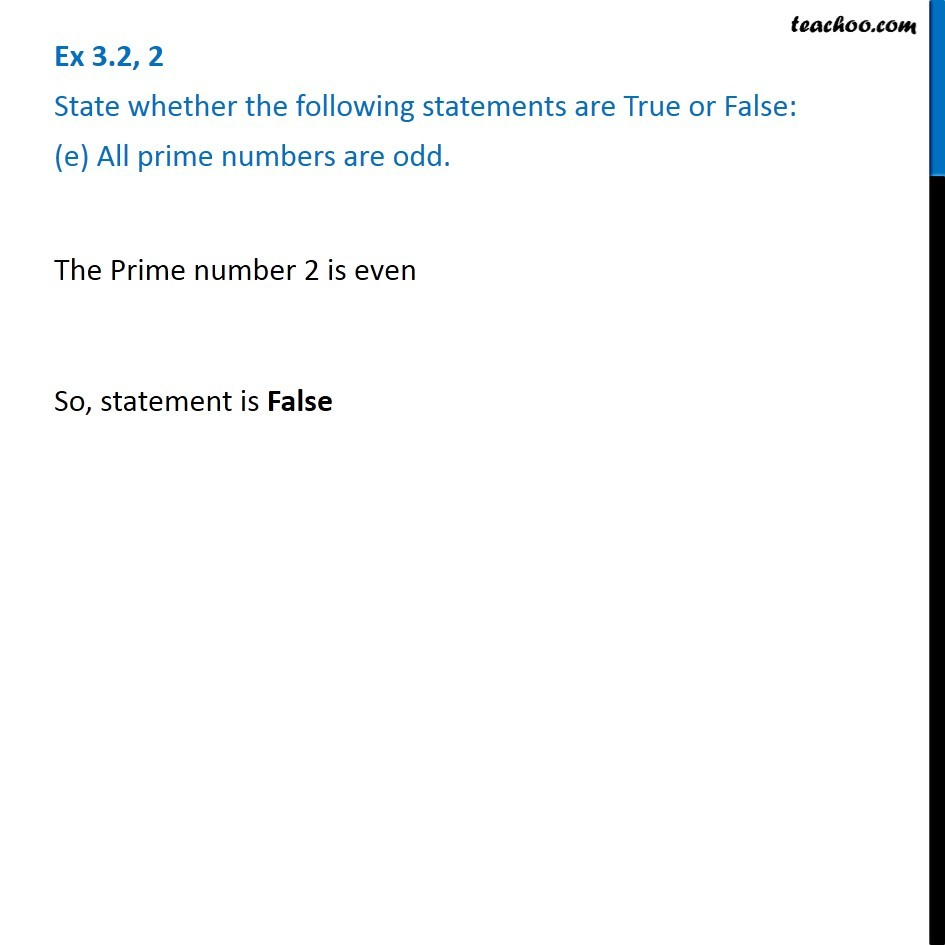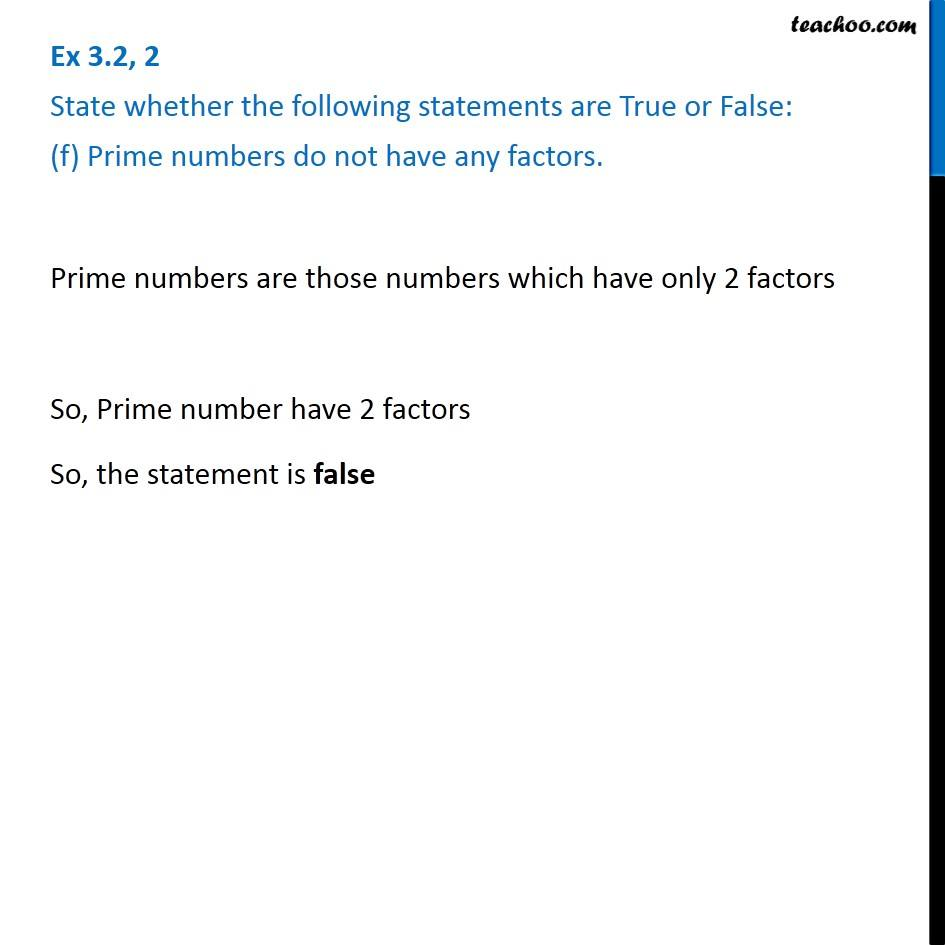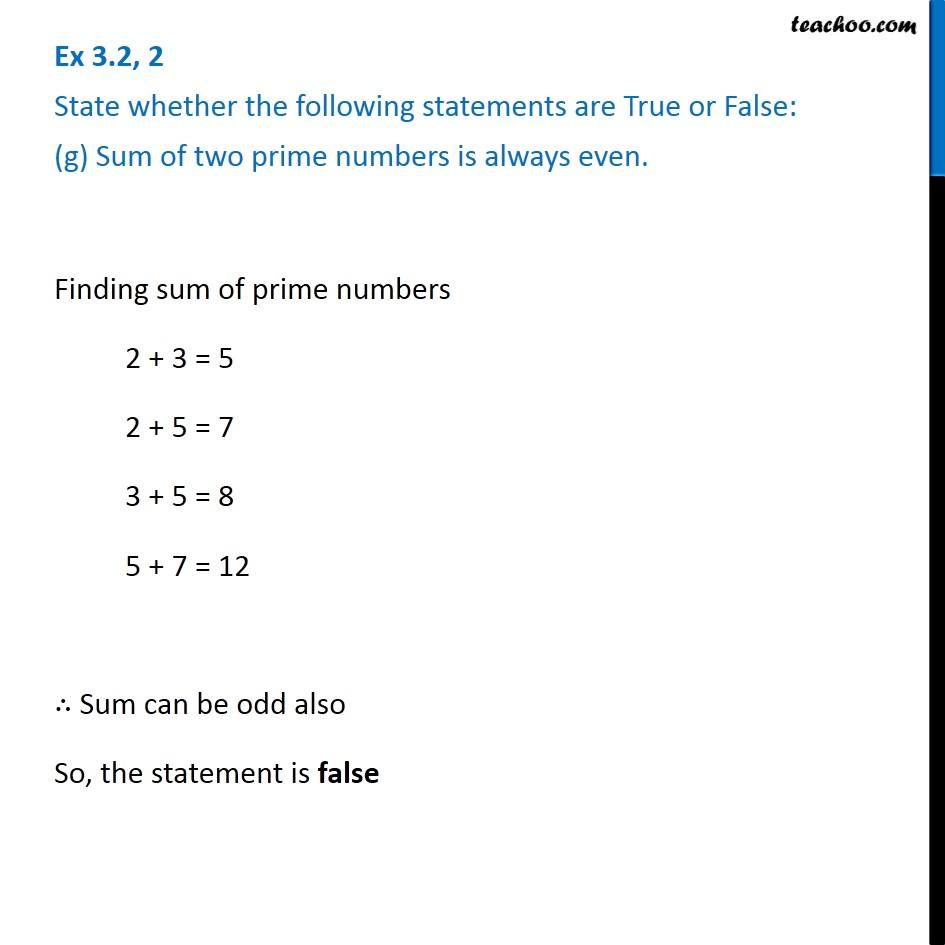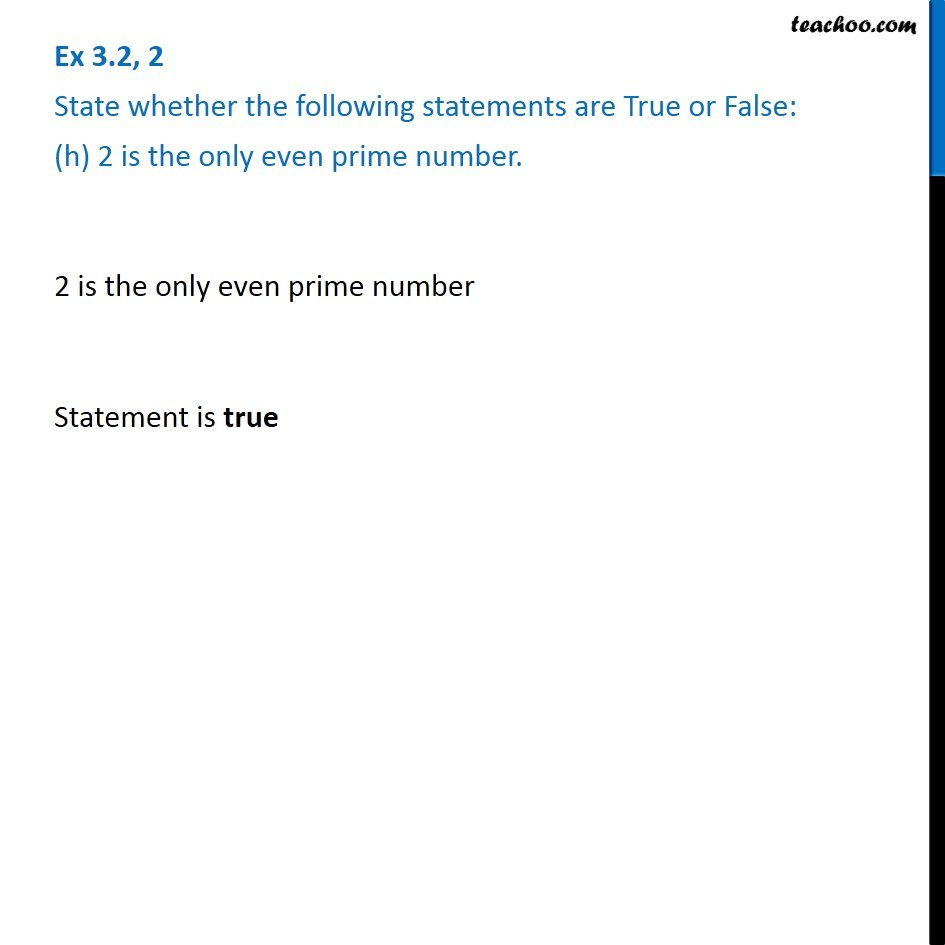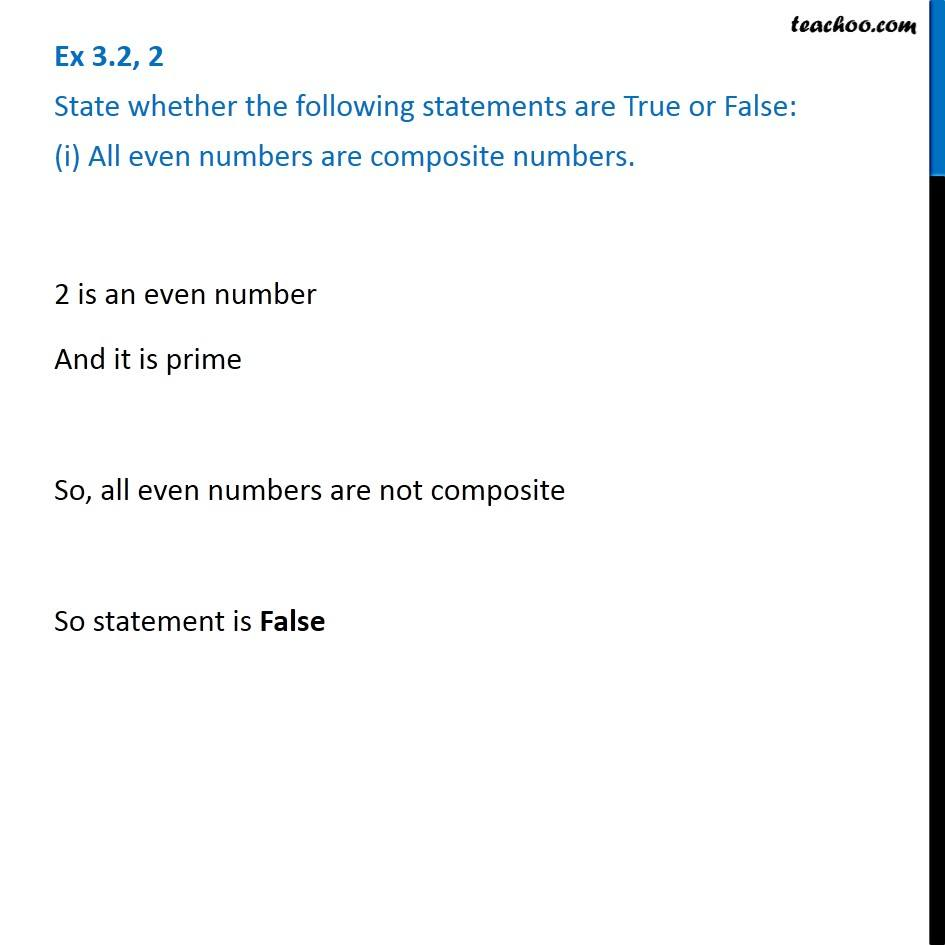1. Chapter 3 Class 6 Playing with Numbers
2. Concept wise
3. Sum of prime numbers

Transcript

Ex 3.2, 2 State whether the following statements are True or False: (a) The sum of three odd numbers is even. Odd numbers are 1, 3, 5, 7, 9, 11, 13, 15, …. Taking any 3 odd numbers and adding them 1 + 3 + 5 = 9 5 + 7 + 9 = 21 11 + 9 + 7 = 27 So sum of three odd number is always odd So, the statement is false Ex 3.2, 2 State whether the following statements are True or False: (b) The sum of two odd numbers and one even number is even. Taking any 2 odd numbers and 1 even number and adding them 1 + 3 + 2 = 6 5 + 7 + 4 = 16 9 + 13 + 6 = 28 So sum of 2 odd numbers and 1 even number is always even So, the statement is true Ex 3.2, 2 State whether the following statements are True or False: (c) The product of three odd numbers is odd. Taking any 3 odd numbers and multiplying them 1 × 3 × 5 = 15 1 × 3 × 7 = 21 3 × 5 × 7 = 105 The Product of three odd numbers is always odd So, the statement is true Ex 3.2, 2 State whether the following statements are True or False: (d) If an even number is divided by 2, the quotient is always odd. Taking any even number and divide it by 2 4/2 = 2 6/2 = 3 8/2 = 4 So, quotient can also be even So, the statement is false Ex 3.2, 2 State whether the following statements are True or False: (e) All prime numbers are odd. The Prime number 2 is even So, statement is False Ex 3.2, 2 State whether the following statements are True or False: (f) Prime numbers do not have any factors. Prime numbers are those numbers which have only 2 factors So, Prime number have 2 factors So, the statement is false Ex 3.2, 2 State whether the following statements are True or False: (g) Sum of two prime numbers is always even. Finding sum of prime numbers 2 + 3 = 5 2 + 5 = 7 3 + 5 = 8 5 + 7 = 12 ∴ Sum can be odd also So, the statement is false Ex 3.2, 2 State whether the following statements are True or False: (h) 2 is the only even prime number. 2 is the only even prime number Statement is true Ex 3.2, 2 State whether the following statements are True or False: (i) All even numbers are composite numbers. 2 is an even number And it is prime So, all even numbers are not composite So statement is False Ex 3.2, 2 State whether the following statements are True or False: (j) The Product of two even numbers is always even. Taking any two even numbers 2 × 4 = 8 4 × 6 = 24 8 × 10 = 80 They are all even So Product of two even number is always even So statement is True

Sum of prime numbers